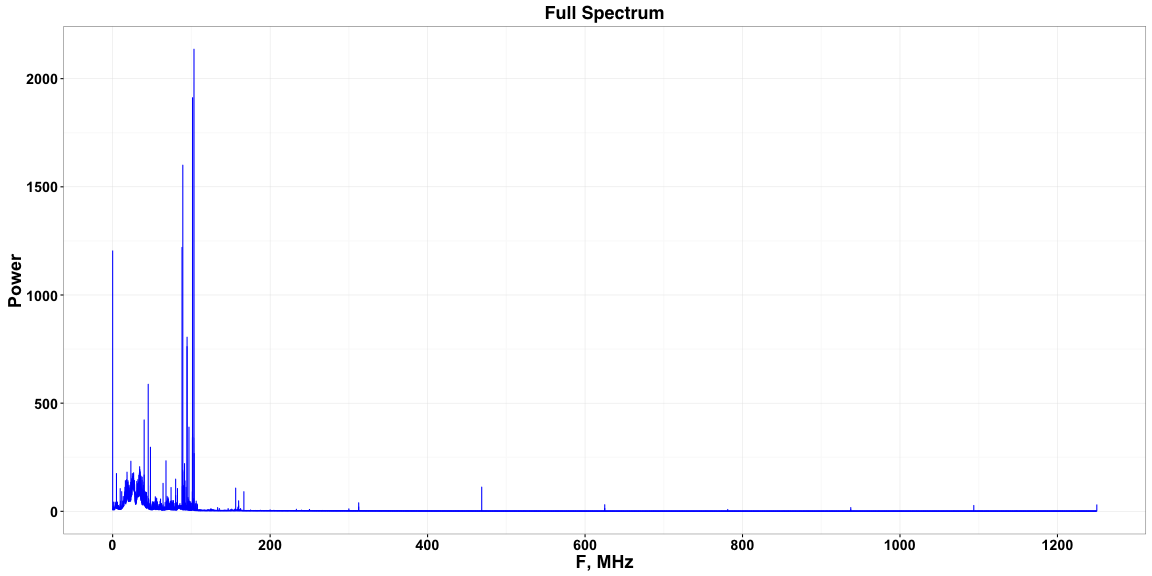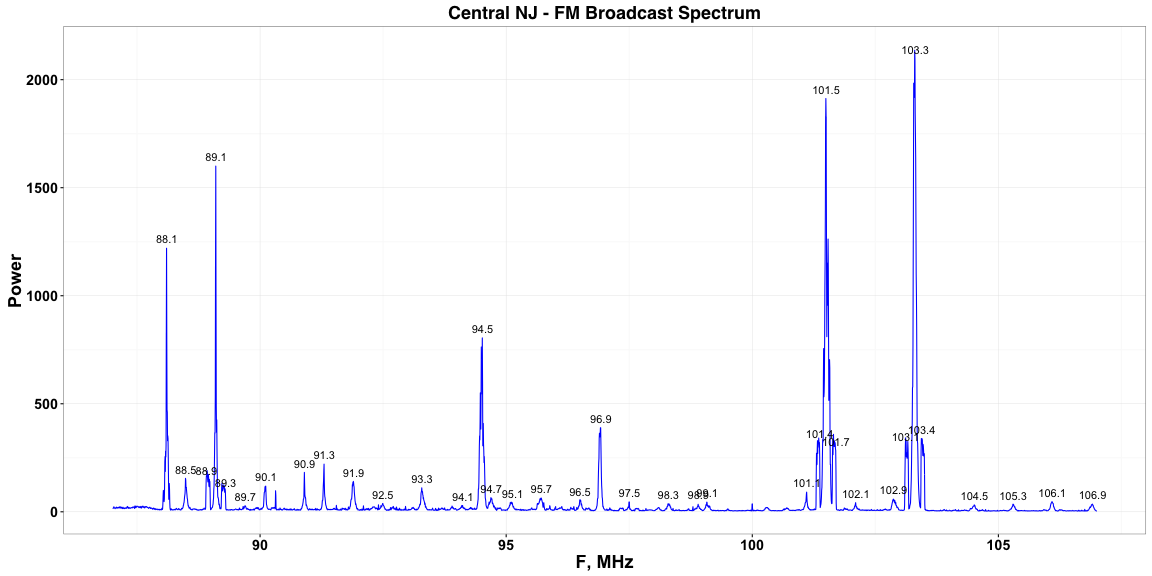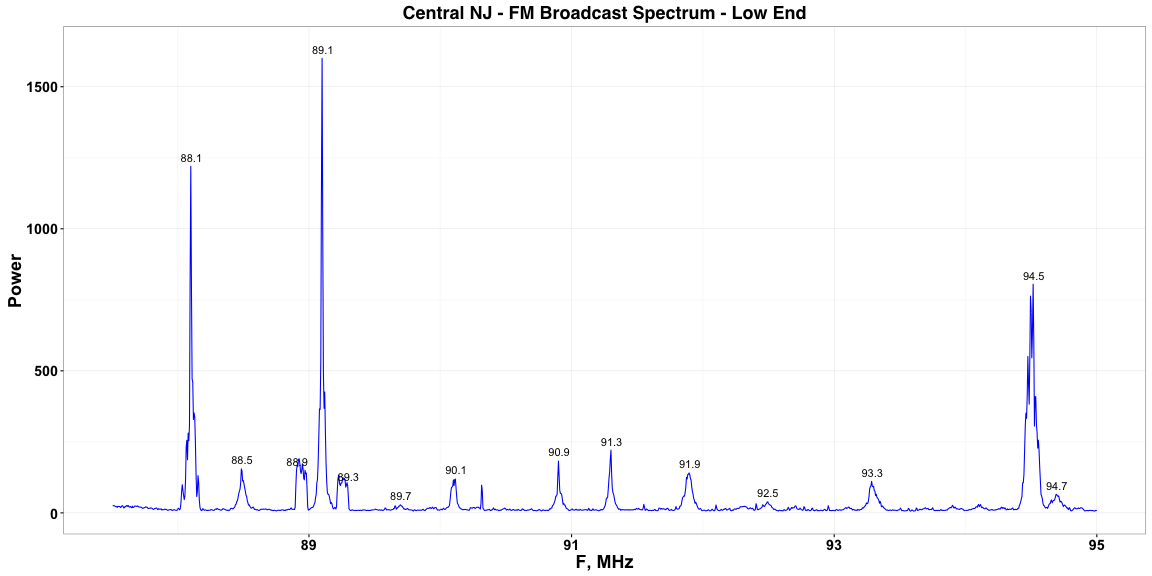## Oscilloscope Based Spectrum Analysis: Acquisition and Long FFT of Waveforms in R

### Jon Meek - November 2016

Do you find the maximum number of FFT points your oscilloscope can handle too limiting? If so, you can do better by transferring the waveform(s) to your PC.

The data acquisition code below is for Keysight scopes with a LAN interface. I plan to test it with the USB interface as well. The code might be adapted to other oscilloscope brands.

## Data Acquisition from Oscilloscope

The code below is available in the oscilloscopeTools repository at https://github.com/meekj/oscilloscopeTools as file scope_comm.R

The oscilloscopeTools repository also contains a small Perl program to save waveforms to ASCII files and simple R code to plot the data from the ASCII files.

``````##
## Demonstration of Oscilloscope Control and Data Acquisition in Pure R
##

## Use IDE such as RStudio or ESS to run blocks of code, or just paste into R session

## Tested on Keysight DSO-X 3024T with Linux and MacOS

# Copyright (C) 2016  Jon Meek

# This program is free software: you can redistribute it and/or modify
# the Free Software Foundation, either version 3 of the License, or
# (at your option) any later version.

# This program is distributed in the hope that it will be useful,
# but WITHOUT ANY WARRANTY; without even the implied warranty of
# MERCHANTABILITY or FITNESS FOR A PARTICULAR PURPOSE.  See the
# GNU General Public License for more details.

# You should have received a copy of the GNU General Public License
# along with this program.  If not, see <http://www.gnu.org/licenses/>.

## \$Id: scope_comm.R,v 1.7 2016/11/20 03:35:35 meekj Exp \$

## The functions below will eventually be part of a R package, likely to be called oscilloscopeR

scopeConnect <- function(addr, port) { # Returns connection object, be sure to close when finished
socketConnection(host = addr, port, timeout=2, open = "r+b", blocking = FALSE)
}

scopeConnectBlocking <- function(addr, port) { # Use for line oriented I/O, not for waveform data
socketConnection(host = addr, port, timeout=2, open = "r+b", blocking = TRUE)
}

scopeGetSingleWaveform <- function(connection, channel, points, Debug = FALSE) {

loop_sleep <- 0.01 #  Sleep a few ms in read loops to give data time to arrive

writeLines("WAVEFORM:FORMAT BYTE", connection)        # Later switch to WORD
flush(connection)
writeLines("WAVeform:POINts:MODE RAW", connection)    # Needed to get more than 62500 points
flush(connection)

num_points_string <- paste('WAVEFORM:POINTS' ,points) # Number of points requested, you might get fewer
writeLines(num_points_string, connection)
flush(connection)

channel_string <- paste('WAVEFORM:SOURCE CHANNEL', channel, sep ='') # Select scope channel
writeLines(channel_string, connection)
flush(connection)

writeLines("WAVEFORM:PREAMBLE?", connection)          # Request the preamble string
flush(connection)

wave_preamble <- NULL
i <- 0
while (length(wave_preamble) == 0) { # Handle non-blocking connection where result can be delayed
i <- i + 1
wave_preamble <- append(wave_preamble, char_read) # Should all be in a single read, but just in case...
Sys.sleep(loop_sleep)
}
if (Debug) {cat(paste("final wave_preamble: ", i, wave_preamble), "\n")}

wave_preamble_items <- unlist(strsplit(wave_preamble, '[,\n]')) # Comma separated, LF terminated

wave_format     <- as.integer(wave_preamble_items) # Unpack the preamble string
wave_type       <- as.integer(wave_preamble_items)
wave_num_points <- as.integer(wave_preamble_items)
wave_count      <- as.integer(wave_preamble_items)
wave_xincrement <- as.numeric(wave_preamble_items)
wave_xorigin    <- as.numeric(wave_preamble_items)
wave_xreference <- as.integer(wave_preamble_items)
wave_yincrement <- as.numeric(wave_preamble_items)
wave_yorigin    <- as.numeric(wave_preamble_items)
wave_yreference <- as.integer(wave_preamble_items)

writeLines("WAVEFORM:DATA?", connection)    # Request waveform data
flush(connection)

i <- 0
while (length(buffer_header) == 0) {        # Get the header, which we ignore for now, the Perl utility uses it
i <- i + 1
Sys.sleep(loop_sleep)
}

buffer <- NULL
i <- 0
while (length(buffer) < wave_num_points) {  # Get the waveform data, looping until we have it all
i <- i + 1
Sys.sleep(loop_sleep)
}
if (Debug) {cat(paste("final buffer read loops: ", i, length(buffer), "\n\n"))}
buffer <- buffer[-length(buffer)] # Drop last element, which seems to be LF

# Return a data frame
data.frame(list(t = wave_xorigin + wave_xincrement * (1:wave_num_points - wave_xreference),
y = wave_yorigin + wave_yincrement * (buffer - wave_yreference) ))
}

## Sample usage - Setup
##
ScopePort <- 5025         # For Keysight

## Example: Get Instrument ID and exit
##
con <- scopeConnectBlocking(ScopeIP, ScopePort) # Blocking connection for getting single line
writeLines("*IDN?", con)
flush(con)
close(con)
ScopeID

## Example: Get Waveform Data from Channel 1 into a Data Frame and Exit
##
AcqPoints <- 10000 # Number of waveform points to request
wf1 <- NULL
con <- scopeConnect(ScopeIP, ScopePort)
wf1 <- scopeGetSingleWaveform(con, 1, AcqPoints)
close(con)
str(wf1) # Look at data frame contents

## Example: Get Waveform Data and Print Some Debug Info
##
wf1 <- NULL
con <- scopeConnect(ScopeIP, ScopePort)
wf1 <- scopeGetSingleWaveform(con, 1, AcqPoints, Debug = TRUE)
close(con)

## Plot the waveform
##
library(ggplot2) # The standard for plotting, IMHO. Now included in the tidyverse package.

ggplot(wf1) + geom_line(aes(x = t * 1e9, y = y), color = 'blue')  + xlab('t, ns')

## Example: PC Side FFT Loop with Frequency Domain Signal Averaging - Do higher resolution FFTs than the scope will do
##
## Random length wire on Channel 1, 50 ohm input impedance, 20 us/div timebase, Single mode.

library(dplyr)      # Data wrangling standard, included in tidyverse package
library(fftwtools)  # Interface to fftw3
library(e1071)      # For Hanning window generation

AcqPoints <- 500000 # 500k points for nice frequency domain resolution
Title <- 'Central NJ - FM Spectrum'

DisplayStart <-  87 # Mhz FM broadcast range, likely something to see here
DisplayEnd   <- 110 # Mhz

wf1 <- NULL
con <- scopeConnect(ScopeIP, ScopePort)

j <- 5 # Number of acquisition loops and frequency domain spectra to average

count <- 0
while (j > 0) {
j <- j - 1
count <- count + 1

writeLines("DIGitize CHANnel1", con)           # Hardcoded channel for testing
flush(con)

wf1 <- scopeGetSingleWaveform(con, 1, AcqPoints, Debug = TRUE) # Get the waveform data
str(wf1) # Display summary of data to verify actual number of points, etc
#    p <- ggplot(wf1) + geom_line(aes(x = t * 1e9, y = y), color = 'blue')  + xlab('t, ns') # Plot raw data
#    print(p)

if (count == 1) {                                       # Compute Hanning window data on the first pass
Hanning <- hanning.window(length(wf1\$y))
}
sfft <- fftw(wf1\$y * Hanning, HermConj=0)               # Compute FFT with Hanning apodization
pwr <- sqrt(Re(sfft) * Re(sfft) + Im(sfft) *  Im(sfft)) # Compute power spectrum

str(pwr)                                                # Look at data on each pass

if (count == 1) {                                       # Compute the x / frequency axis on first pass
pwr_sum <- pwr
TimePerChannel <- wf1\$t - wf1\$t
NyquistFrequency <- (1 / (2*TimePerChannel))
FrequencyPerChannel <- NyquistFrequency / (length(pwr_sum) - 1)
f <- seq(from = 0, by = FrequencyPerChannel, length = length(pwr_sum))

pwr_spectrum <- data.frame(list(F = f, Pwr = pwr_sum))
pwr_spectrum\$F <- pwr_spectrum\$F / 1e6              # Convert to MHz for this example

} else {
pwr_sum <- pwr_sum + pwr                            # Signal average
pwr_spectrum\$Pwr <- pwr_sum                         # Put current average into the data frame
}

pTitle <- paste(Title, ' -- ', count, 'averages')       # Plot the current averaged power spectrum
display_data <- pwr_spectrum %>% filter(F >= DisplayStart & F <= DisplayEnd)
p <- ggplot(display_data) + geom_line(aes(x = F, y = Pwr), color = 'blue') +
xlab('F, MHz') + ggtitle(pTitle) # + scale_y_log10()
print(p)

Sys.sleep(2)                                            # Delay so that we can observe the spectrum on each loop
}
close(con)

str(pwr_spectrum)

## Peak Detection and Plotting Demonstation
##
library(wmtsa) # For peak detection, uses MASS which overloads dplyr's select

DisplayStart <-  87 # Mhz Full FM broadcast range
DisplayEnd   <- 107 # Mhz

DisplayStart <-  87.5 # Mhz Lower end of FM broadcast range
DisplayEnd   <-  95.0 # Mhz

display_data <- pwr_spectrum %>% filter(F >= DisplayStart & F <= DisplayEnd)

SNRmin <- 1 # Find some of the larger peaks
W <- wavCWT(display_data\$Pwr)
z <- wavCWTTree(W)
pk <- wavCWTPeaks(z, snr.min = SNRmin, length.min = 2, noise.fun = 'sd', noise.span = 2)
str(pk)

f0 <- display_data\$F # Channel to frequency conversion
df <- display_data\$F - display_data\$F

Peaks <- data.frame(list(F = f0 + df * pk\$x, Height = pk\$y, Chan = pk\$x)) # Put peaks in a data frame
Peaks                                                                     # List the peaks

Title <- 'Central NJ - FM Broadcast Spectrum'

HeightThreshold <- 20 # Arbitrary, and manually set, should select Top N and watch distance between labels
Peaks <- Peaks %>% filter(Height > HeightThreshold)
Peaks # List the peaks

label_offset <- 0.02 * max(display_data\$Pwr) # Position peak labels just above peak top

ggplot(display_data) + geom_line(aes(x = F, y = Pwr), color = 'blue') +
xlab('F, MHz') + ylab('Power') + ggtitle(Title) +
geom_text(data = Peaks, aes(x = F, y = Height +
label_offset, label = sprintf('%0.1f', F)))

## Save plot to PDF
OutFile <- '/n2/r-reports/fm2.pdf'
ggsave(OutFile, dpi=150, height = 8.5, width = 11)

## Save plot to PNG
OutFile <- '/n2/r-reports/fm2.png'
ggsave(OutFile, dpi=150, height = 4, width = 8) # Labels too close to peak tops with 4 x 8

## Write frequency domain data to a file
File <- '~/lab/R/data/fd-5avg-dualfs-1.dat'
write.table(pwr_spectrum, File, quote=FALSE) # Space separated data file
``````

## Use R to Read and Display Previously Saved Frequency Domain Data

``````RCSid <- '\$Id: scope_long_fft.Rmd,v 1.2 2016/11/29 15:52:17 meekj Exp \$'

library(ggplot2)

FigureWidth  <- 16
FigureHeight <- 8

## A custom ggplot2 theme that is decent for HTML reports
theme_jm2 <- theme_bw() +
theme(
plot.title  = element_text(size = rel(1.5), family = 'Helvetica', face = 'bold'),
axis.title  = element_text(size = rel(1.5), colour = "black", face = 'bold'),
axis.text.x = element_text(size = rel(1.5), lineheight = 0.9, colour = "black", vjust = 1, face = 'bold'),
axis.text.y = element_text(size = rel(1.5), lineheight = 0.9, colour = "black", hjust = 1, face = 'bold'),
legend.text = element_text(size = rel(1.3)),
plot.title = element_text(lineheight=.8, face="bold", size = 10),
strip.text.y = element_text(size = rel(1.7), colour = "black", face = 'bold') # Enhance readability of facet labels
)

File <- '~/lab/R/data/scope-fanfare-5Gs-50ohm-fd-25avg-1.dat' # A previously acquired frequency domain spectrum

head(pwr_spectrum, 10) # Inspect the data
``````
``````##        F         Pwr
## 1  0.000 1205.644740
## 2  0.005  604.576512
## 3  0.010   14.471696
## 4  0.015   10.273220
## 5  0.020    9.412489
## 6  0.025    8.872968
## 7  0.030   11.026974
## 8  0.035   11.235450
## 9  0.040    8.621293
## 10 0.045    8.716225
``````
``````tail(pwr_spectrum, 5)
``````
``````##               F       Pwr
## 249997 1249.980  1.637999
## 249998 1249.985  1.973246
## 249999 1249.990  2.075267
## 250000 1249.995 15.562661
## 250001 1250.000 31.706677
``````

## Simple FFT Example Result

``````Title <- 'Full Spectrum'

ggplot(pwr_spectrum) + geom_line(aes(x = F, y = Pwr), color = 'blue') +
scale_x_continuous(breaks = seq(0, max(pwr_spectrum\$F), 200), minor_breaks = seq(0, max(pwr_spectrum\$F), 100)) +
xlab('F, MHz') + ylab('Power') + ggtitle(Title) + theme_jm2
````````````num_channels <- nrow(pwr_spectrum)            # Get information on the data set
df   <- pwr_spectrum\$F - pwr_spectrum\$F
maxf <- pwr_spectrum\$F[num_channels]
``````

The full spectrum above consists of 250001 channels with maximum frequency 1250 MHz and channel width 0.005 MHz. The scope's analog bandwidth is 200 MHz. The peak at 468.8 MHz is real, but it's height is obviously attenuated. The higher frequency peaks are probably real as well, but we have only verified frequency measurement up to 500 MHz using a frequency synthesizer with a random length wire on the output. The power axis is arbitrary units, for now.

## Look at the FM Broadcast Spectrum, with Peak Labels

The code below is essentially the same as shown above.

``````suppressMessages(library(wmtsa)) # For peak detection, uses MASS which overloads dplyr's select

DisplayStart <-  87 # Mhz  Select a subset of the data to plot
DisplayEnd   <- 107 # Mhz
display_data <- pwr_spectrum %>% filter(F >= DisplayStart & F <= DisplayEnd)

SNRmin <- 1 # Find some of the larger peaks
W <- wavCWT(display_data\$Pwr)
z <- wavCWTTree(W)
pk <- wavCWTPeaks(z, snr.min = SNRmin, length.min = 2, noise.fun = 'sd', noise.span = 2)

f0 <- display_data\$F # Channel to frequency conversion
df <- display_data\$F - display_data\$F

Peaks <- data.frame(list(F = f0 + df * pk\$x, Height = pk\$y, Chan = pk\$x)) # Put peaks in a data frame

Title <- 'Central NJ - FM Broadcast Spectrum'

HeightThreshold <- 20 # Arbitrary, and manually set, should select Top N and watch distance between labels
Peaks <- Peaks %>% filter(Height > HeightThreshold)
Peaks # List the peaks
``````
``````##          F     Height Chan
## 1   88.105 1219.89893  220
## 2   88.490  154.84498  297
## 3   88.910  148.19561  381
## 4   89.105 1600.12358  420
## 5   89.300   94.87124  459
## 6   89.700   28.43083  539
## 7   90.120  118.91966  623
## 8   90.905  182.50272  780
## 9   91.305  220.69756  860
## 10  91.900  140.81735  979
## 11  92.495   38.52238 1098
## 12  93.290  110.99895 1257
## 13  94.120   29.14563 1423
## 14  94.520  804.97777 1503
## 15  94.695   65.47024 1538
## 16  95.130   41.25435 1625
## 17  95.715   63.90940 1742
## 18  96.500   50.26462 1899
## 19  96.925  389.06756 1984
## 20  97.505   46.85099 2100
## 21  98.295   37.52423 2258
## 22  98.905   34.90362 2380
## 23  99.085   44.32167 2416
## 24 101.110   91.13943 2821
## 25 101.370  320.60330 2873
## 26 101.500 1912.81970 2899
## 27 101.700  284.10272 2939
## 28 102.105   42.41482 3020
## 29 102.870   58.51169 3173
## 30 103.115  304.94909 3222
## 31 103.310 2098.31708 3261
## 32 103.440  339.43002 3287
## 33 104.515   32.18975 3502
## 34 105.300   34.78832 3659
## 35 106.095   47.35505 3818
## 36 106.915   35.70935 3982
``````
``````label_offset <- 0.02 * max(display_data\$Pwr) # Position peak labels just above peak top

ggplot(display_data) + geom_line(aes(x = F, y = Pwr), color = 'blue') +
xlab('F, MHz') + ylab('Power') + ggtitle(Title) +
geom_text(data = Peaks, aes(x = F, y = Height +
label_offset, label = sprintf('%0.1f', F))) + theme_jm2
``````## A Closer Look at the Low End

``````DisplayStart <-  87.5 # Mhz  Select a subset of the data to plot
DisplayEnd   <-  95   # Mhz
display_data <- pwr_spectrum %>% filter(F >= DisplayStart & F <= DisplayEnd)

SNRmin <- 1 # Find some of the larger peaks
W <- wavCWT(display_data\$Pwr)
z <- wavCWTTree(W)
pk <- wavCWTPeaks(z, snr.min = SNRmin, length.min = 2, noise.fun = 'sd', noise.span = 2)

f0 <- display_data\$F # Channel to frequency conversion
df <- display_data\$F - display_data\$F

Peaks <- data.frame(list(F = f0 + df * pk\$x, Height = pk\$y, Chan = pk\$x)) # Put peaks in a data frame

Title <- 'Central NJ - FM Broadcast Spectrum - Low End'

HeightThreshold <- 20
Peaks <- Peaks %>% filter(Height > HeightThreshold)
Peaks # List the peaks
``````
``````##         F     Height Chan
## 1  88.105 1219.89893  120
## 2  88.490  154.84498  197
## 3  88.910  148.19561  281
## 4  89.105 1600.12358  320
## 5  89.300   94.87124  359
## 6  89.700   28.43083  439
## 7  90.120  118.91966  523
## 8  90.905  182.50272  680
## 9  91.305  220.69756  760
## 10 91.900  140.81735  879
## 11 92.495   38.52238  998
## 12 93.290  110.99895 1157
## 13 94.520  804.97777 1403
## 14 94.695   65.47024 1438
``````
``````label_offset <- 0.02 * max(display_data\$Pwr)

ggplot(display_data) + geom_line(aes(x = F, y = Pwr), color = 'blue') +
xlab('F, MHz') + ylab('Power') + ggtitle(Title) +
geom_text(data = Peaks, aes(x = F, y = Height +
label_offset, label = sprintf('%0.1f', F))) + theme_jm2
``````\$Id: scope_long_fft.Rmd,v 1.2 2016/11/29 15:52:17 meekj Exp \$